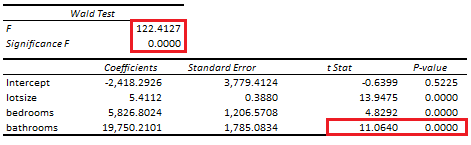# Omitted Variable Bias: Wald Test

Last Update: February 21, 2022

Omitted Variable Bias is when one or more linear regression independent variables were incorrectly omitted from model equation. This can be tested through Wald test which adds independent variables to model equation and evaluates whether they explain dependent variable. If added independent variables explain dependent variable, then they were incorrectly omitted from model equation.

As example, we can fit a three-variable restricted multiple linear regression with formula . Then, as example again, we can fit a four-variable unrestricted multiple linear regression by adding independent variable  with formula . Next, we can do Wald test with null hypothesis that independent variable  coefficient  is equal to zero with formula . If null hypothesis is rejected, then independent variable  was incorrectly omitted from restricted multiple linear regression (1). Notice that unrestricted multiple linear regression (2) can include more independent variables. Also, notice that null hypothesis test (3) can de done with an F-test or a chi-square test.

Below, we find an example of unrestricted multiple linear regression of house price explained by its lot size, number of bedrooms and bathrooms coefficients and number of bathrooms omitted variable Wald test .Table 1. Microsoft Excel® unrestricted multiple linear regression of house price explained by its lot size, number of bedrooms and bathrooms coefficients and number of bathrooms omitted variable Wald test.

Courses

My online courses are hosted at Teachable website.

For more details on this concept, you can view my Linear Regression Courses.

 Data Description: Sales prices of houses sold in the city of Windsor, Canada, during July, August and September, 1987.

Original Source: Anglin, P., and Gencay, R. (1996). Semiparametric Estimation of a Hedonic Price Function. Journal of Applied Econometrics, 11, 633–648.

Source: AER R Package HousePrices Object. Christian Kleiber and Achim Zeileis. (2008). Applied Econometrics with R. Springer-Verlag, New York.

+